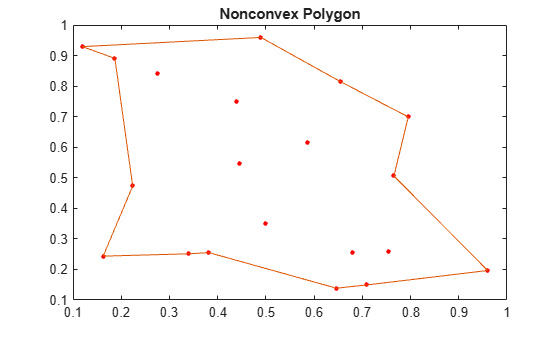## Types of Region Boundaries

### Convex Hulls vs. Nonconvex Polygons

The convex hull of a set of points in N-D space is the smallest convex region enclosing all points in the set. If you think of a 2-D set of points as pegs in a peg board, the convex hull of that set would be formed by taking an elastic band and using it to enclose all the pegs.

```rng('default') x = rand(20,1); y = rand(20,1); plot(x,y,'r.','MarkerSize',10) hold on k = convhull(x,y); plot(x(k),y(k)) title('The Convex Hull of a Set of Points') hold off```A convex polygon is a polygon that does not have concave vertices, for example:

```x = rand(20,1); y = rand(20,1); k = convhull(x,y); plot(x(k),y(k)) title('Convex Polygon')```You can also create a boundary of a point set that is nonconvex. If you "vacuum pack" the convex hull from above, you can enclose all of the points in a nonconvex polygon with concave vertices:

```k = boundary(x,y,0.9); plot(x(k),y(k)) title('Nonconvex Polygon')```The convex hull has numerous applications. You can compute the upper bound on the area bounded by a discrete point set in the plane from the convex hull of the set. The convex hull simplifies the representation of more complex polygons or polyhedra. For instance, to determine whether two nonconvex bodies intersect, you could apply a series of fast rejection steps to avoid the penalty of a full intersection analysis:

• Check if the axis-aligned bounding boxes around each body intersect.

• If the bounding boxes intersect, you can compute the convex hull of each body and check intersection of the hulls.

If the convex hulls did not intersect, this would avoid the expense of a more comprehensive intersection test.

While convex hulls and nonconvex polygons are convenient ways to represent relatively simple boundaries, they are in fact specific instances of a more general geometric construct called the alpha shape.

### Alpha Shapes

The alpha shape of a set of points is a generalization of the convex hull and a subgraph of the Delaunay triangulation. That is, the convex hull is just one type of alpha shape, and the full family of alpha shapes can be derived from the Delaunay triangulation of a given point set.

```rng(4) x = rand(20,1); y = rand(20,1); plot(x,y,'r.','MarkerSize',20) hold on shp = alphaShape(x,y,100); plot(shp) title('Convex Alpha Shape') hold off```Unlike the convex hull, alpha shapes have a parameter that controls the level of detail, or how tightly the boundary fits around the point set. The parameter is called alpha or the alpha radius. Varying the alpha radius from 0 to `Inf` produces a set of different alpha shapes unique for that point set.

```plot(x,y,'r.','MarkerSize',20) hold on shp = alphaShape(x,y,.5); plot(shp) title('Nonconvex Alpha Shape') hold off```Varying the alpha radius can sometimes result in an alpha shape with multiple regions, which might or might not contain holes. However, the `alphaShape` function in MATLAB® always returns regularized alpha shapes, which prevents isolated or dangling points, edges, or faces.

```plot(x,y,'r.','MarkerSize',20) hold on shp = alphaShape(x,y); plot(shp) title('Alpha Shape with Multiple Regions') hold off```